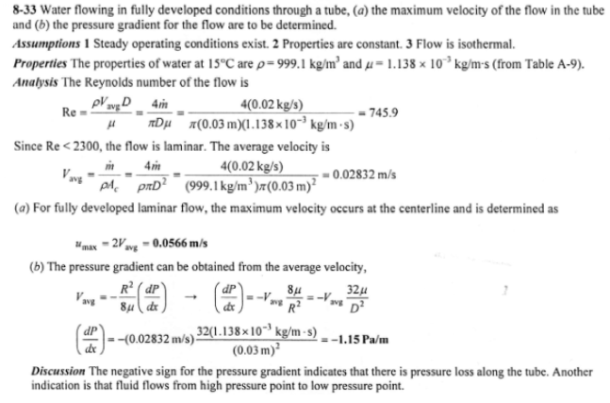heat and mass transfer problems engineering equations heat and mass transfer school homework engineering heat and mass transfer formulas heat and mass transfer solutions to heat and mass transfer problems full solution engineering problem solution heat and mass transfer math problems engineering equations heat and mass transfer school homework engineering solutions to heat and mass transfer formulas heat problems mass problem solutions to transfer problems full solution heat and mass transfer
heat and mass transfer problems engineering equations heat and mass transfer school homework engineering heat and mass transfer formulas heat and mass transfer solutions to heat and mass transfer problems full solution engineering problem solution heat and mass transfer math problems engineering equations heat and mass transfer school homework engineering solutions to heat and mass transfer formulas heat problems mass problem solutions to transfer problems full solution heat and mass transfer
Highalphabet Home Page heat and mass transfer problem solutions Heat and Mass Transfer Page
Water is flowing in fully developed conditions through a 3-cm diameter smooth tube with a mass flow rate of 0.02 kg/s at 15 C. Determine (a) the maximum velocity of the flow in the tube and (b) the pressure gradient for the flow.Water is flowing in fully developed conditions through a 3-cm diameter smooth tube with a mass flow rate of 0.02 kg/s at 15 C. Determine (a) the maximum velocity of the flow in the tube and (b) the pressure gradient for the flow.